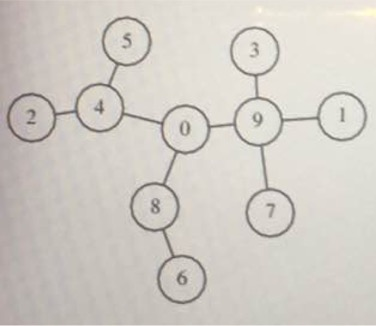2017-01-22 04:19

# c语言小白求解一道算法题，能编写运行出结果立即采纳给分9处于距离1;
0,3,7处于距离2;
8,4在距离3;
2，5，6距离为4。

struct Results {

int ＊ A;
int N.
};
（A代表需要返回的数组，N代表数组的大小）

struct Results solution（int T [ ]，int M），

T  = 9 T  = 1 T  = 4
T  = 9 T  = 0 T  = 4
T  = 8 T  = 9 T  = 0
T  = 1

M是在范围[1 ... 100,000]内的整数;

• 点赞
• 写回答
• 关注问题
• 收藏
• 邀请回答

#### 7条回答默认 最新

•Kolamu 2017-01-22 06:19
已采纳

我看是对的！

``````struct Results solution(int T[],int M)
{
int cap = 0;
int* res = (int*)malloc((M-1)*sizeof(int));
for(int i=0;i<M-1;i++)
{
res[i] = 0;
}
for(int i=0;i<M;i++)
{
int v = T[i];
int n = i;

int index = 0;
if(n == v)
{
continue;
}
while(v != n)
{
index++;
n = v;
v = T[v];
}

res[index-1]++;
}

Results* rs = (Results *)malloc(sizeof(Results));
rs->A = res;
rs->N = M-1;

return *rs;
}
``````
点赞 打赏 评论
•wild84 2017-01-22 05:00

mark，同是小白，等着看答案。我想用java实现是不是简单点。

点赞 打赏 评论
•奔跑的小鱼儿 2017-01-22 05:09

你又来了，我又来了，搞不懂你

点赞 打赏 评论
•Kolamu 2017-01-22 05:20

A和N分别表示啥呀？

点赞 打赏 评论
•奔跑的小鱼儿 2017-01-22 05:23

你要是在做算法题，刷题库之类的直接找个学长要答案不就好了吗

点赞 打赏 评论
•很久没有写C程序了，结构体还有函数调用这些都忘了，不过还是有一些思路，这里给朋友分享一下。
主要思路：
1、求得每点到首都的距离
2、对距离进行统计

我把第1点的关键代码贴出来，看看能不能给楼主一点启发

`````` #include "stdafx.h"
#include "stdio.h"

int main(int argc, char* argv[])
{

int T = {9,1,4,9,0,4,8,9,0,1};
int D = {0};

int i , p;
//遍历每点
for(i = 0; i<10; i++)
{
//求点到首都的距离
for(p = i; p != T[p]; p = T[p])
{
D[i] = D[i]++;
}
}

//输出0--10到首都的距离
//for(i = 0; i<10; i++){
//  printf("%d\n" , D[i]);
//}

return 0;
}
``````
点赞 打赏 评论
•小傅傅阿明 2017-01-22 09:39

#include
#include
struct Results {
int* A;
int N;
};
struct Results solution(int T[], int M) {
//创建result并初始化。
struct Results result = { (int*)malloc((M - 1) * sizeof(int)), (M - 1) };
//定义一个temp数组，尺寸和传进来的那个T一样。
//temp数组是用来储存城市和首都的距离。比如temp的值为2表示城市0和首都距离为2。
int* temp = (int*)malloc(M * sizeof(int));
int i, j;
//将temp数组初始化。
for (i = 0; i < M; i++) {
temp[i] = 0;
}
//将result的成员A数组初始化。
for (i = 0; i < result.N; i++) {
result.A[i] = 0;
}
//开始填充temp数组。这里的循环中i代表城市标号。
for (i = 0; i < M; i++) {
j = i;
//如果城市标号j和T[j]不一致，那么就朝首都前进一个单位。
while (j != T[j]) {
//每前进一个单位就要记录一次。
temp[i]++;
j = T[j];
}
}
//统计距离。存入result的成员A数组里面。
for (i = 0; i < M; i++) {
if (temp[i]) {
result.A[--temp[i]]++;
}

}
return result;
}
//测试
int main() {
int T = { 9, 1, 4, 9, 0, 4, 8, 9, 0, 1 };
struct Results results = solution(T, 10);
for (int i = 0; i < results.N; i++) {
printf("%d ", results.A[i]);
}
return 0;
}

点赞 打赏 评论